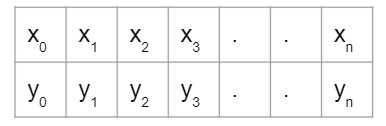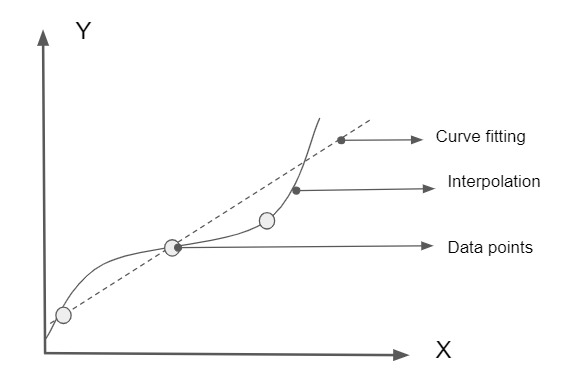## Interpolation

In Interpolation we will construct a curve using the given data points and we make implicit assumptions that the data points are accurate and distinct

or

Interpolation is the process of estimating the value of y=f(x) corresponding to the value of x.

for every value of y there is a corresponding value of x

Here x is an independent variable and is a dependent variable

## Curve fitting

curve fitting is applied to the data that usually contains scatter. these scatters are usually formed by measurement errors in the data

## Diagramatic representation

Data set :